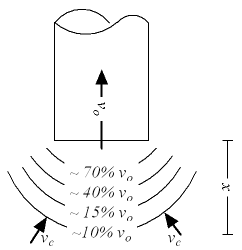## Capture Air Velocity solver#### Smaller Outlets

air flow (m3/s, ft3/s)
distance (m, ft)

#### Larger Outlets

air flow (m3/s, ft3/s)
distance (m, ft)
area (m2, ft2)

### Capture Air Velocity formula

vc = q / (4 π x2) = vo A / (12 x2) = vo π d2 / (48 x2).

where

A = duct area (m2, ft2)

q = air volume flow (m3/s, ft3/s)

vo = air velocity in the exhaust outlet opening (m/s, ft/s)

vc = capture air velocity in distance x from exhaust outlet (m/s, ft/s)

x = distance from exhaust outlet (m, ft)

d = diameter of exhaust outlet (m, ft)

To larger exhaust outlets it should be modified to

vc = vo A / (A + 10 x2) = q / (A + 10 x2)

Thinkcalculator.com provides you helpful and handy calculator resources.